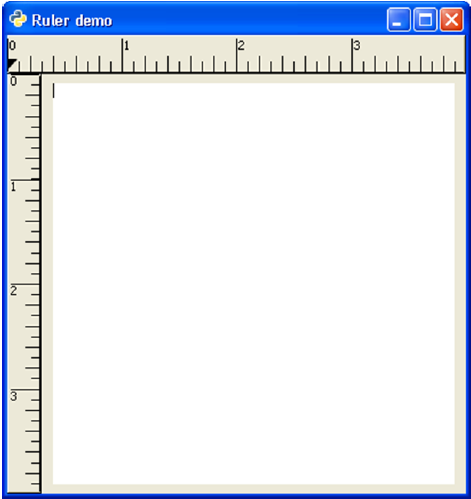# PyGTK - Ruler Class

This is a base class for horizontal (gtk.Hruler) and vertical (gtk.Vruler) rulers that are useful to show mouse pointer's position in window. A small triangle in the ruler indicates the location of pointer.

Ruler objects are created with their respective constructors −

```hrule = gtk.Hruler()
vrule = gtk.Vruler()
```

The following gtk.Ruler class methods are available for both the derived classes −

• Ruler.set_metric() − This sets the measurement unit. The predefined metric constants are: gtk.PIXELS (default), gtk.INCHES and gtk.CENTIMETERS

• Ruler.set_range() − This sets the lower and upper bounds, position and maximum size of ruler.

In the example given below, the horizontal and vertical rulers are placed above and to the left of a gtk.TextView widget.

The measurement of horizontal ruler is in pixels. Its minimum and maximum values are 0 and 400 respectively. It is placed in the upper row of a gtk.VBox.

```hrule = gtk.HRuler()
hrule.set_metric(gtk.PIXELS)
hrule.set_range(0, 4,0,0.5)
vbox.pack_start(hrule)
```

The lower row of Vbox contains an HBox. A vertical ruler and a TextView widget, in which a multi-line text can be entered, is packed.

```vrule=gtk.VRuler()
vrule.set_metric(gtk.PIXELS)
vrule.set_range(0, 4, 10, 0.5)
hbox.pack_start(vrule)
```

## Example

Observe the following code −

```import gtk
class PyApp(gtk.Window):

def __init__(self):
super(PyApp, self).__init__()

self.set_title("Ruler demo")
self.set_size_request(400,400)
self.set_position(gtk.WIN_POS_CENTER)

vbox = gtk.VBox()
tv = gtk.TextView()
tv.set_size_request(350,350)

hrule = gtk.HRuler()
hrule.set_metric(gtk.PIXELS)
hrule.set_range(0, 4,0,0.5)

vbox.pack_start(hrule)
hbox = gtk.HBox()
vrule = gtk.VRuler()

vrule.set_metric(gtk.PIXELS)
vrule.set_range(0, 4, 10, 0.5)

hbox.pack_start(vrule)
halign = gtk.Alignment(0.5, 0.5, 0, 0)

hbox.pack_start(halign, False, True, 10)